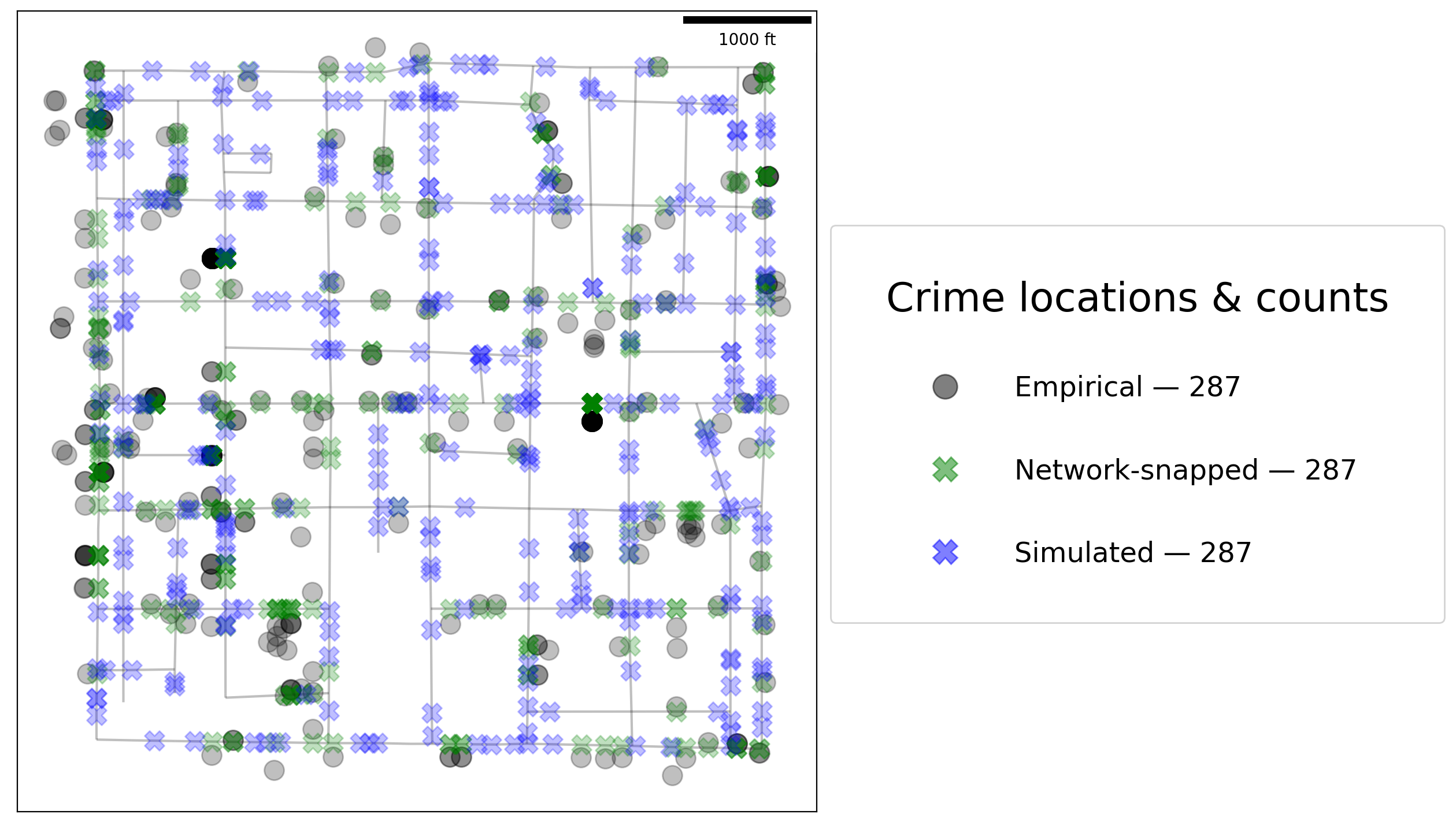If any part of this notebook is used in your research, please cite with the reference found in README.md.

# Network point pattern attributes¶

## Demonstrating network point pattern representation¶

Author: James D. Gaboardi jgaboardi@gmail.com

This notebook is an basic walk-through for:

1. Exploring the attributes of network objects and point patterns

2. Generating observation counts per network link

3. Simulating a point pattern

:

%config InlineBackend.figure_format = "retina"

:

%load_ext watermark
%watermark

Last updated: 2022-04-16T17:02:06.319507-04:00

Python implementation: CPython
Python version       : 3.10.4
IPython version      : 8.2.0

Compiler    : Clang 12.0.1
OS          : Darwin
Release     : 21.4.0
Machine     : x86_64
Processor   : i386
CPU cores   : 8
Architecture: 64bit


:

import geopandas
import libpysal
import matplotlib
import matplotlib_scalebar
from matplotlib_scalebar.scalebar import ScaleBar
import numpy
import pandas
import shapely
from shapely.geometry import Point
import spaghetti

%matplotlib inline
%watermark -w
%watermark -iv

/Users/the-gaboardi/spaghetti/spaghetti/network.py:36: FutureWarning: The next major release of pysal/spaghetti (2.0.0) will drop support for all libpysal.cg geometries. This change is a first step in refactoring spaghetti that is expected to result in dramatically reduced runtimes for network instantiation and operations. Users currently requiring network and point pattern input as libpysal.cg geometries should prepare for this simply by converting to shapely geometries.
warnings.warn(f"{dep_msg}", FutureWarning)

Watermark: 2.3.0

matplotlib         : 3.5.1
numpy              : 1.21.6
libpysal           : 4.6.2
matplotlib_scalebar: 0.8.0
pandas             : 1.4.2
spaghetti          : 1.6.5+44.g17b88a0.dirty
json               : 2.0.9
geopandas          : 0.10.2
shapely            : 1.8.0



## Instantiating a spaghetti.Network object¶

### Instantiate the network from a .shp file¶

:

ntw = spaghetti.Network(in_data=libpysal.examples.get_path("streets.shp"))


## 1. Allocating observations (snapping points) to a network:¶

A network is composed of a single topological representation of network elements (arcs and vertices) to which point patterns may be snapped.

:

pp_name = "crimes"
pp_shp = libpysal.examples.get_path("%s.shp" % pp_name)
ntw.snapobservations(pp_shp, pp_name, attribute=True)
ntw.pointpatterns

:

{'crimes': <spaghetti.network.PointPattern at 0x10bf57220>}


### Attributes for every point pattern¶

1. dist_snapped dict keyed by point id with the value as snapped distance from observation to network arc

:

ntw.pointpatterns[pp_name].dist_snapped

:

221.58676169738433

1. dist_to_vertex dict keyed by pointid with the value being a dict in the form {node: distance to vertex, node: distance to vertex}

:

ntw.pointpatterns[pp_name].dist_to_vertex

:

{161: 83.70599311338093, 162: 316.8274480625799}

1. npoints point observations in set

:

ntw.pointpatterns[pp_name].npoints

:

287

1. obs_to_arc dict keyed by arc with the value being a dict in the form {pointID:(x-coord, y-coord), pointID:(x-coord, y-coord), … }

:

ntw.pointpatterns[pp_name].obs_to_arc[(161, 162)]

:

{0: (727919.2473619275, 875942.4986759046)}

1. obs_to_vertex list of incident network vertices to snapped observation points

:

ntw.pointpatterns[pp_name].obs_to_vertex

:

161

1. points geojson like representation of the point pattern. Includes properties if read with attributes=True

:

ntw.pointpatterns[pp_name].points

:

{'coordinates': (727913.0000000029, 875720.9999999977), 'properties': [[1, 1]]}

1. snapped_coordinates dict keyed by pointid with the value being (x-coord, y-coord)

:

ntw.pointpatterns[pp_name].snapped_coordinates

:

(727919.2473619275, 875942.4986759046)


## 3. Simulate a point pattern on the network¶

• The number of points must supplied.

• The only distribution currently supported is uniform.

• Generally, this will not be called by the user since the simulation will be used for Monte Carlo permutation.

:

npts = ntw.pointpatterns[pp_name].npoints
npts

:

287

:

sim_uniform = ntw.simulate_observations(npts)
sim_uniform

:

<spaghetti.network.SimulatedPointPattern at 0x16856f4f0>

:

print(dir(sim_uniform))

['__class__', '__delattr__', '__dict__', '__dir__', '__doc__', '__eq__', '__format__', '__ge__', '__getattribute__', '__gt__', '__hash__', '__init__', '__init_subclass__', '__le__', '__lt__', '__module__', '__ne__', '__new__', '__reduce__', '__reduce_ex__', '__repr__', '__setattr__', '__sizeof__', '__str__', '__subclasshook__', '__weakref__', 'dist_to_vertex', 'npoints', 'obs_to_arc', 'obs_to_vertex', 'points', 'snapped_coordinates']


### Extract the simulated points along the network a geopandas.GeoDataFrame¶

:

def as_gdf(pp):
pp = {idx: Point(coords) for idx, coords in pp.items()}
gdf = geopandas.GeoDataFrame.from_dict(
pp, orient="index", columns=["geometry"]
)
gdf.index.name = "id"
return gdf

sim_uniform_gdf = as_gdf(sim_uniform.points)

:

geometry
id
0 POINT (725240.363 876554.280)
1 POINT (726772.962 879414.944)
2 POINT (728612.296 876978.197)
3 POINT (727577.106 877133.996)
4 POINT (726020.596 878468.896)

### Create geopandas.GeoDataFrame objects of the vertices and arcs¶

:

vertices_df, arcs_df = spaghetti.element_as_gdf(
ntw, vertices=ntw.vertex_coords, arcs=ntw.arcs
)


### Create geopandas.GeoDataFrame objects of the actual and snapped crime locations¶

:

crimes = spaghetti.element_as_gdf(ntw, pp_name=pp_name)
crimes_snapped = spaghetti.element_as_gdf(ntw, pp_name=pp_name, snapped=True)


### Helper plotting function¶

:

def plotter():
"""Generate a spatial plot."""

def _patch(_kws, labinfo):
"""Generate a legend patch."""
label = "%s — %s" % tuple(labinfo)
_kws.update({"lw":0, "label":label, "alpha":.5})
return matplotlib.lines.Line2D([], [], **_kws)

def _legend(handles, anchor=(1., .75)):
"""Generate a legend."""
lkws = {"fancybox":True,"framealpha":0.85, "fontsize":"xx-large"}
lkws.update({"bbox_to_anchor": anchor, "labelspacing": 2.})
lkws.update({"title": "Crime locations & counts", "title_fontsize":25})
matplotlib.pyplot.legend(handles=handles, **lkws)

def carto_elements(b):
kw = {"units":"ft", "dimension":"imperial-length", "fixed_value":1000}
b.set(xticklabels=[], xticks=[], yticklabels=[], yticks=[]);

pkws = {"alpha":0.25}
base = arcs_df.plot(color="k", figsize=(9, 9), zorder=0, **pkws)
patches = []
gdfs = [crimes, crimes_snapped, sim_uniform_gdf]
colors, zo = ["k", "g", "b"], [1 ,2 ,3]
markers, markersizes = ["o", "X", "X"], [150, 150, 150]
labels = [["Empirical"], ["Network-snapped"], ["Simulated"]]
iterinfo = list(zip(gdfs, colors, zo, markers, markersizes, labels))
for gdf, c, z, m, ms, lab in iterinfo:
gdf.plot(ax=base, c=c, marker=m, markersize=ms, zorder=z, **pkws)
patch_args = {"marker":m, "markersize":ms/10,"c":c}, lab+[gdf.shape]
patches.append(_patch(*patch_args))
_legend(patches)
carto_elements(base)


### Crimes: empirical, network-snapped, and simulated locations¶

:

plotter()